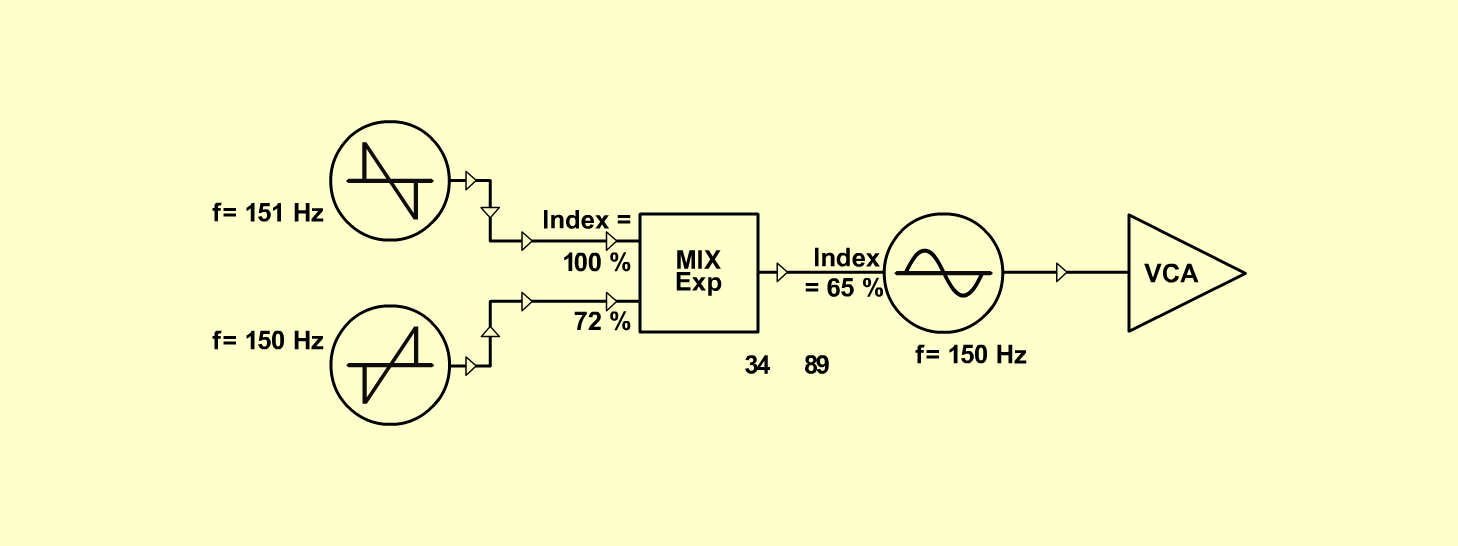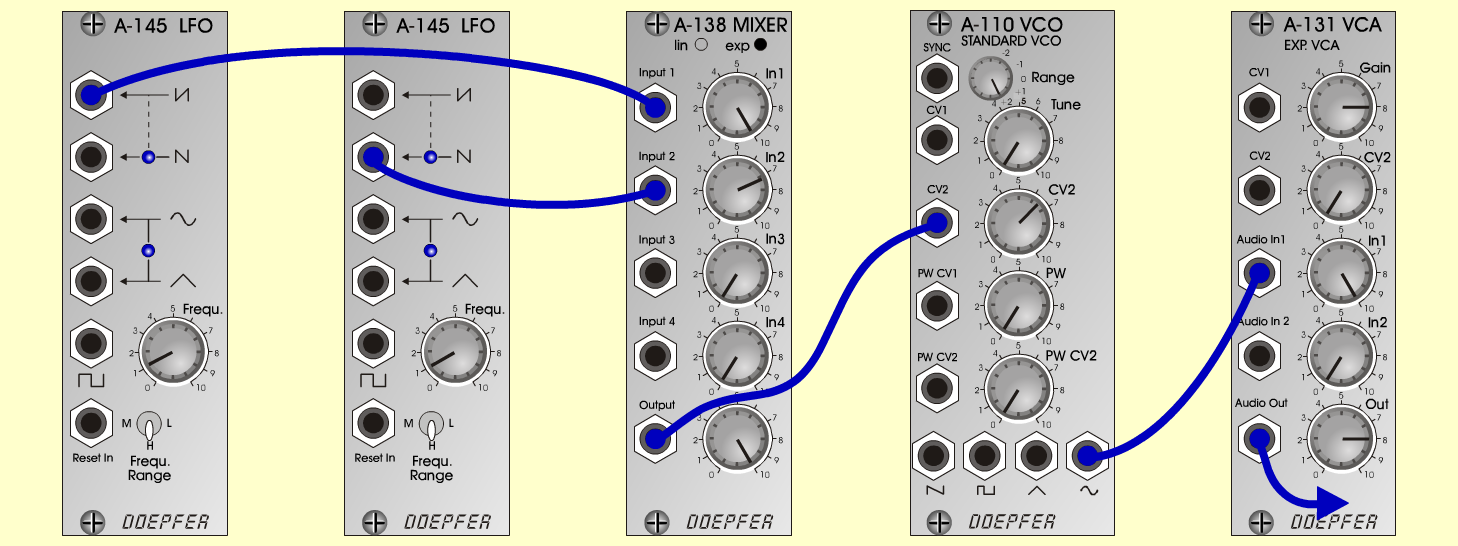ShephardFXD o e p f e r A - 100 Connections: Settings: A-145/1 (Saw down)  <=>  A-138exp (Input 1) A-145/2 (Saw up)  <=>  A-138exp (Input 2) A-138exp (Output)  <=>  A-110 (CV 2) A-110 (Sine)  <=>  A-131 (Audio In) A-145/1 (Frq = 1.1, Range = H) A-145/2 (Frq = 1, Range = H) A-138exp (In 1 = 10, In 2 = 7.2, Out = 10) A-110 (Range = +2, CV 2 = 6.5) A-131 (Gain = 8, Audio In 1 = 10,  Audio Out = 8) Notes: It has to be two separate lfo and their frequency has to be almost the same. As slow beating against each other as possible. Keld Sørensen, January 2002Sound sample Shephard